• Slides: 11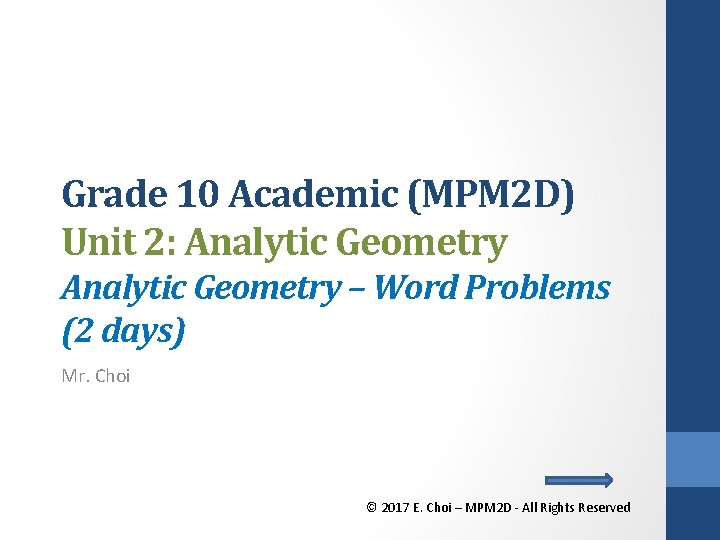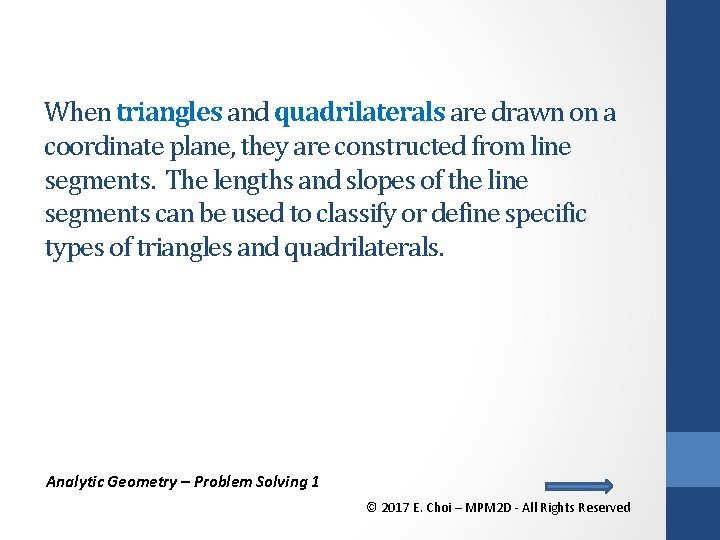When triangles and quadrilaterals are drawn on a coordinate plane, they are constructed from line segments. The lengths and slopes of the line segments can be used to classify or define specific types of triangles and quadrilaterals. Analytic Geometry – Problem Solving 1 © 2017 E. Choi – MPM 2 D - All Rights ReservedTriangles If you know the coordinates of the vertices of a triangle, then You can use the formula for the length of a line segment to determine the lengths of the sides of the triangle, and decide if the triangle is - Scalene (no sides equal), - Isosceles (two sides equal), or - Equilateral (all sides equal) You can use the slopes of the sides to determine if the triangle has a right angle. Analytic Geometry – Problem Solving 1 © 2017 E. Choi – MPM 2 D - All Rights ReservedQuadrilaterals If you know the coordinates of the vertices of a quadrilateral, You can determine the length and slopes of the sides and use the information to classify the quadrilateral as - Parallelogram (both pairs of opposite sides are parallel), - Rectangle (parallelogram and adjacent sides are perpendicular), - Square (rectangle and all sides are same length), - Rhombus (both pairs of opposite sides are parallel and all sides are the same length). Analytic Geometry – Problem Solving 1 © 2017 E. Choi – MPM 2 D - All Rights Reserved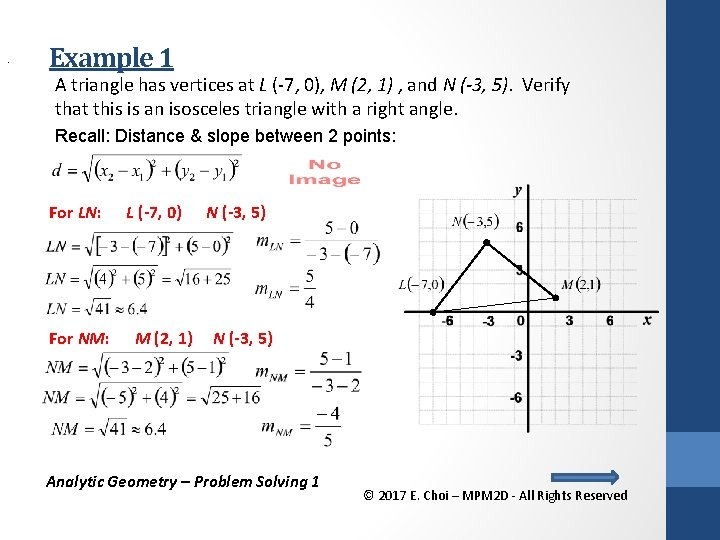. Example 1 A triangle has vertices at L (-7, 0), M (2, 1) , and N (-3, 5). Verify that this is an isosceles triangle with a right angle. Recall: Distance & slope between 2 points: For LN: For NM: L (-7, 0) M (2, 1) N (-3, 5) Analytic Geometry – Problem Solving 1 © 2017 E. Choi – MPM 2 D - All Rights Reserved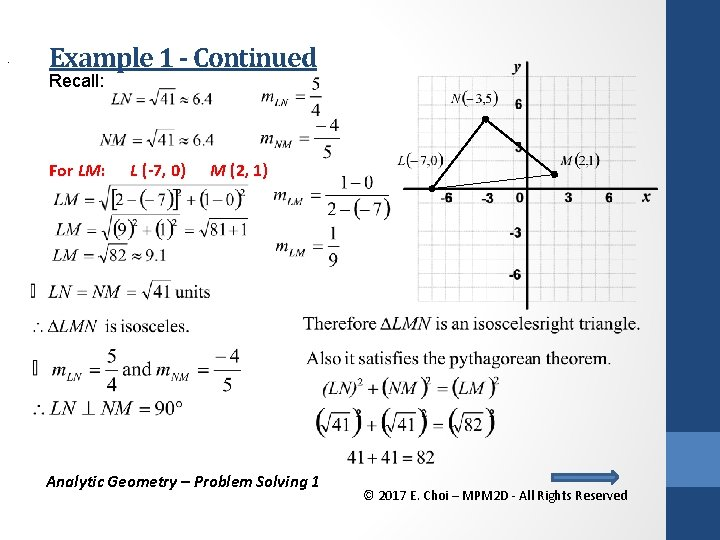. Example 1 - Continued Recall: For LM: L (-7, 0) M (2, 1) Analytic Geometry – Problem Solving 1 © 2017 E. Choi – MPM 2 D - All Rights Reserved. Example 2 An engineer needs to check the “setting out” of the base of a rectangular building before construction work on the foundation begins. Junior engineers have marked out the corners of the building at coordinates P (6, 36), Q (-30, 9) , R (-12, -15) and S (24, 12), where each unit represents 1 m. The building is to be 45 m long and 30 m wide. Check that the given coordinates do mark out a rectangle with dimensions 45 m x 30 m. Recall: Distance & slope between 2 points: Analytic Geometry – Problem Solving 1 © 2017 E. Choi – MPM 2 D - All Rights Reserved. Example 2 - Continued For PQ: P (6, 36) Q (-30, 9) For QR: Q (-30, 9) R (-12, -15) For RS: R (-12, -15) S (24, 12) For SP: S (24, 12) P (6, 36) Analytic Geometry – Problem Solving 1 © 2017 E. Choi – MPM 2 D - All Rights Reserved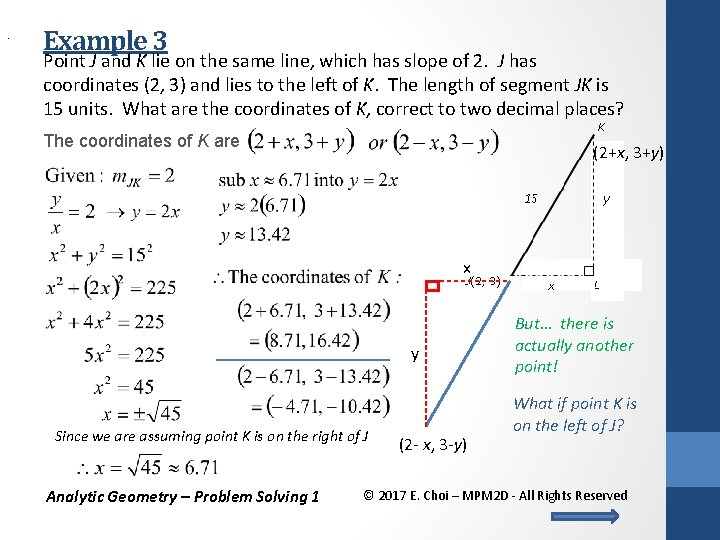. Example 3 Point J and K lie on the same line, which has slope of 2. J has coordinates (2, 3) and lies to the left of K. The length of segment JK is 15 units. What are the coordinates of K, correct to two decimal places? The coordinates of K are (2+x, 3+y) x y Since we are assuming point K is on the right of J Analytic Geometry – Problem Solving 1 (2 - x, 3 -y) But… there is actually another point! What if point K is on the left of J? © 2017 E. Choi – MPM 2 D - All Rights Reserved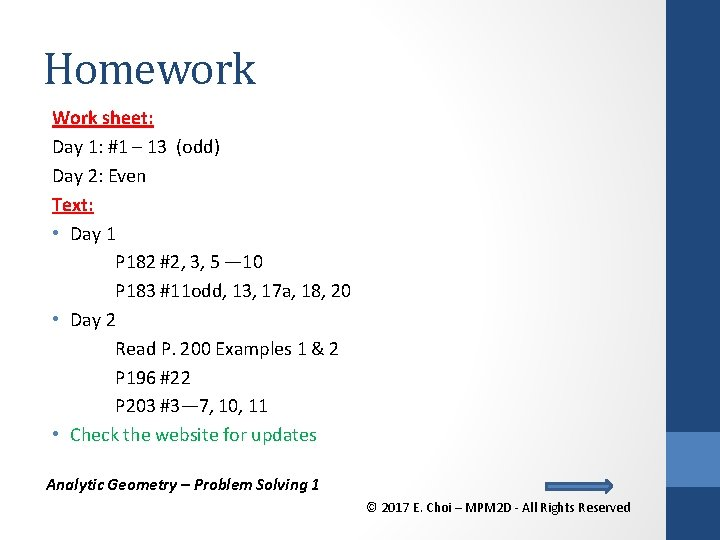Homework Work sheet: Day 1: #1 – 13 (odd) Day 2: Even Text: • Day 1 P 182 #2, 3, 5 — 10 P 183 #11 odd, 13, 17 a, 18, 20 • Day 2 Read P. 200 Examples 1 & 2 P 196 #22 P 203 #3— 7, 10, 11 • Check the website for updates Analytic Geometry – Problem Solving 1 © 2017 E. Choi – MPM 2 D - All Rights Reserved